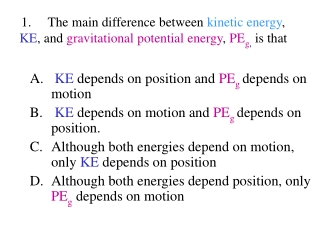Download PresentationKE depends on position and PE g depends on motion

KE depends on position and PE g depends on motion

Download PresentationKE depends on position and PE g depends on motion

- - - - - - - - - - - - - - - - - - - - - - - - - - - E N D - - - - - - - - - - - - - - - - - - - - - - - - - - -
Presentation Transcript

1. 1.     The main difference between kinetic energy, KE, and gravitational potential energy, PEg, is that • KE depends on position and PEgdepends on motion • KE depends on motion and PEg depends on position. • Although both energies depend on motion, only KE depends on position • Although both energies depend position, only PEg depends on motion

2. 2h h 2. Joe raised a box above the ground. If he lifts the same box twice as high, it has • four times the potential energy • twice the potential energy • there is no change in potential energy.

3. 3. As any object free falls, the gravitational potential energy • vanishes • is immediately converted to kinetic energy • is converted into kinetic energy gradually until it reaches the ground

4. 4. A small mass, starting at rest, slides without friction down a rail to a loop-de-loop as shown. Will the ball make it to the top of the loop? A. Yes B. No

5. B. Mass at maximum height D. Spring with no mass attached A. C. Mass at minimum height A spring is hanging from a fixed wire as in the first picture on the left. Then a mass is hung on the spring and allowed to oscillate freely (with no friction present). Answers A-D show different positions of the mass as it was oscillating. 5. Where does the spring have maximum elastic potential energy?

6. B. Mass at maximum height D. Spring with no mass attached A. C. Mass at minimum height A spring is hanging from a fixed wire as in the first picture on the left. Then a mass is hung on the spring and allowed to oscillate freely (with no friction present). Answers A-D show different positions of the mass as it was oscillating. 6. Where is thegravitational potential energythe least?

7. B. Mass at maximum height D. Spring with no mass attached A. C. Mass at minimum height 7. Where is thekinetic energyzero? A spring is hanging from a fixed wire as in the first picture on the left. Then a mass is hung on the spring and allowed to oscillate freely (with no friction present). Answers A-D show different positions of the mass as it was oscillating.

8. B. Mass at maximum height D. Spring with no mass attached A. C. Mass at minimum height 8. Where is the elastic potential energy zero? A spring is hanging from a fixed wire as in the first picture on the left. Then a mass is hung on the spring and allowed to oscillate freely (with no friction present). Answers A-D show different positions of the mass as it was oscillating.

9. 9. When the hanging mass is stationary, the force that a very hard spring exerts on the hanging mass is … the force that is exerted on the same hanging mass by a very soft spring? • greater than B. equal to C. Less than